Include Top

# Exponentially Weighted Moving Average (EWMA) Chart

1. Click SigmaXL > Templates & Calculators > Control Chart Templates > Time Weighted > EWMA. This template is also located at SigmaXL > Control Charts > Control Chart Templates > Time Weighted > EWMA.

2. Open Montgomery Table 9.1.xlsx (Sheet 1 tab). This is simulated data from Montgomery, D.C. (2013), Introduction to Statistical Quality Control, Seventh Ed., Wiley, pp. 415. Table 9.1. Samples 1 to 20 are drawn from a random normal distribution with population mean µ = 10 and population standard deviation σ = 1. Samples 21 to 30 have a mean = 11 and standard deviation = 1, so the process has shifted by 1 sigma and is “out-of-control”. While this is a small shift in process mean, it is something that we want to be able to detect and correct as quickly as possible.

3.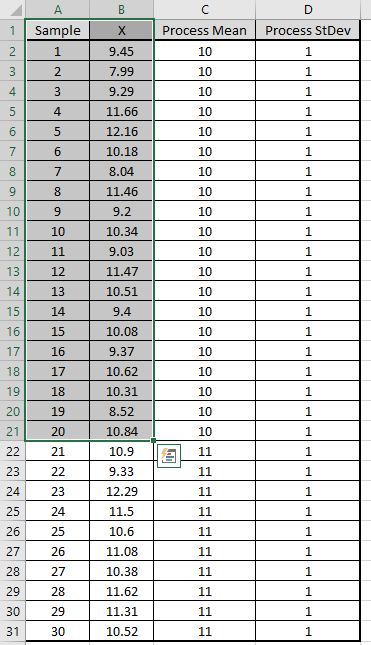4. Copy cells A1:B21 and Paste Values to the template at A1. Use the default Weight (Lambda) = 0.1, K = 2.7. Specify Historical Mean = 10 and Historical StDev = 1 as shown:

5.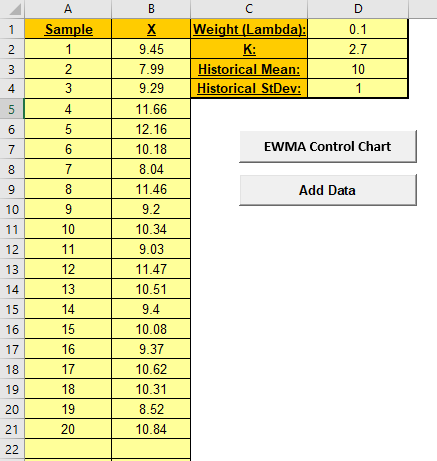6. Click the EWMA Control Chart button to create the EWMA Control Chart:

7.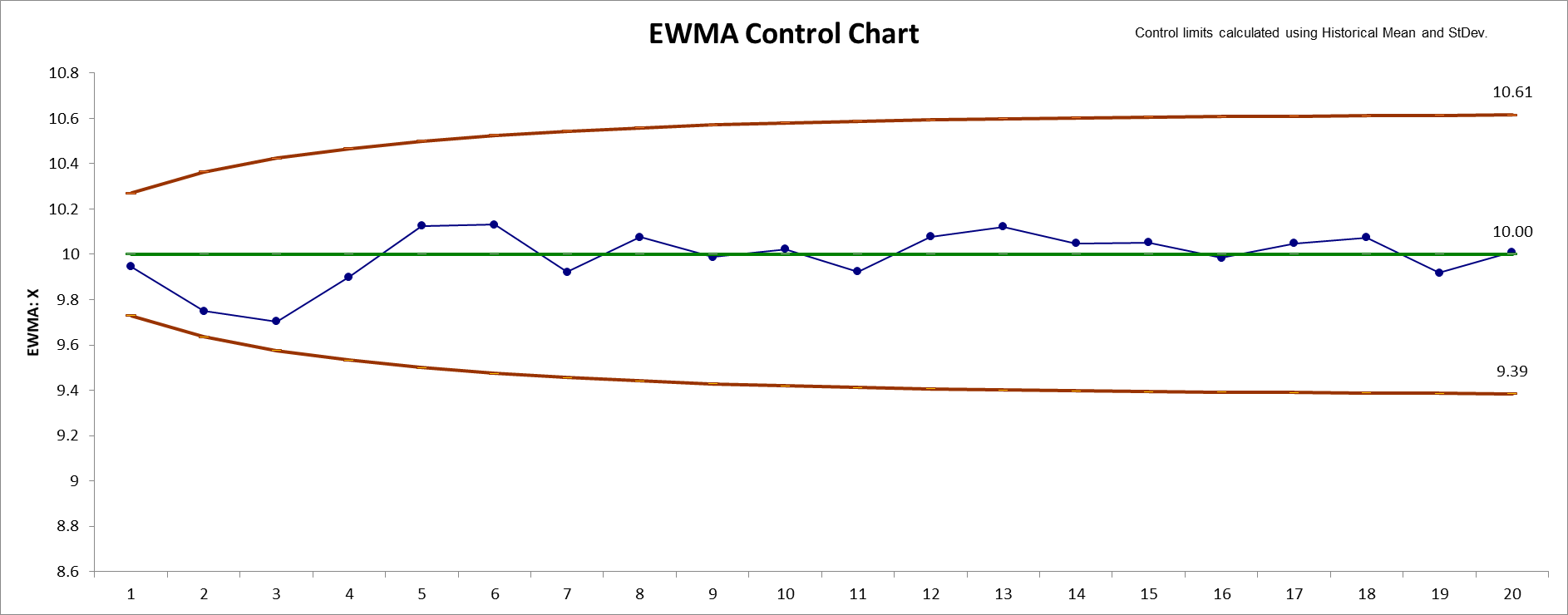8. This confirms that the process is “in-control”.

9. Switch back to Montgomery Table 9.1.xlsx (Sheet 1 tab). Select and copy cells A22: B31 as shown. This is the data with shifted mean.

10.11. Switch to the EWMA template and Paste Values to cell A22 as shown.

12.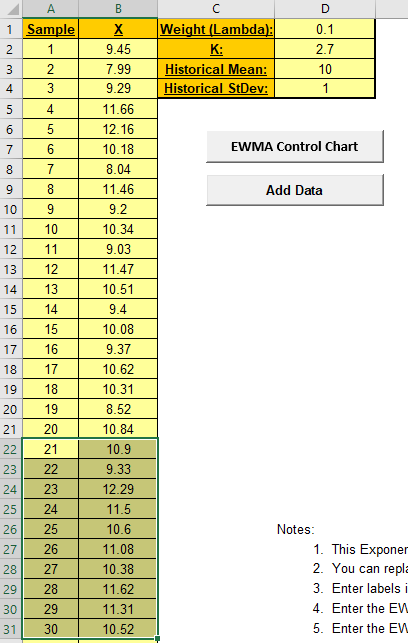13. Click the Add Data button to add the new data to the EWMA Control Chart:

14.15. This confirms that the process is now “out-of-control” with signals at samples 29 and 30. This matches Figure 9.7 in Montgomery.

The EWMA control chart uses weighted averages, where the weights decrease exponentially as observations come from further in the past with the smallest weights associated with the oldest observations.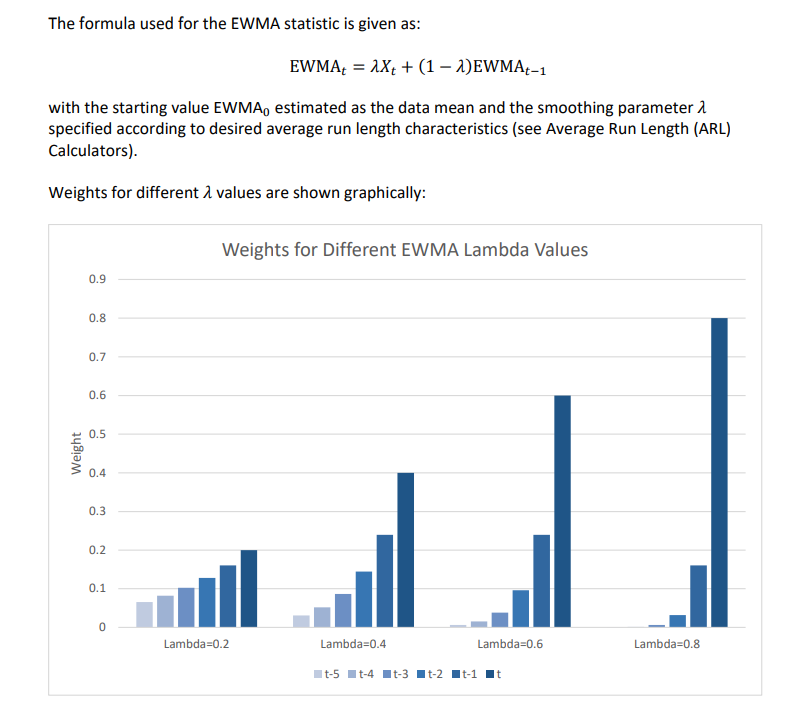# Template Notes and References:

1. This Exponentially Weighted Moving Average (EWMA) Control Chart template should be used with continuous data. The data must be in chronological time-sequence order.

2. You can replace the X-Axis Label and Data column headings with any headings that you wish. Enter your data in the Data column.

3. Enter labels in X-Axis Label column. Labels can be Date, Time, Name, or other text information. These labels are optional and will appear on the horizontal X-Axis of the EWMA Control Chart.

4. Enter the EWMA Weight (Lambda) in cell D1. This is a value between 0 and 1 and controls the amount of influence that previous observations have on the current EWMA statistic. A value near 1 puts almost all weight on the current observation, making it resemble a Shewhart chart. For values near 0, a small weight is applied to almost all of the past observations, so the EWMA chart performance is similar to that of a CUSUM chart.

5. Enter the EWMA K StDev multiplier in cell D2. This is a value between 2 and 4. This is also referred to as L, but SigmaXL uses K to avoid confusion with Lambda.

6. Weight (Lambda) and K values affect the Average Run Length (ARL) characteristics. To determine optimal EWMA parameter values and calculate ARL, click SigmaXL > Templates and Calculators > Control Chart Templates > Average Run Length (ARL) Calculators > EWMA ARL.

7. Historical Mean (D3) and Historical StDev (D4) are optional. Enter values if process mean and standard deviation are known.

8. Click the EWMA Control Chart button to create an EWMA Control Chart.

9. After the control chart has been created and additional new data entered into the Data column, click the Add Data button to add the data to the existing chart. Control limits will be calculated using the original chart mean and stdev or specified Historical Mean/StDev.

10. Add Data should only be used if there are at least 20 observations in the original chart, or Historical Mean and StDev have been specified.

11. Weight (Lambda) and K parameters are dynamic. If they are modified, the chart will automatically update with the new parameters. However, they should be selected prior to creating the EWMA chart. Data values and out-of-control formatting are refreshed only when the buttons are used.

12. Reference: Montgomery, D.C. (2013), Introduction to Statistical Quality Control, Seventh Ed., Wiley, pp. 433-438.

# Web Demos

Our CTO and Co-Founder, John Noguera, regularly hosts free Web Demos featuring SigmaXL and DiscoverSim